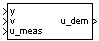# 1D Self-Conditioned [A(v),B(v),C(v),D(v)]

Implement gain-scheduled state-space controller in self-conditioned form depending on one scheduling parameter

•Libraries:
Aerospace Blockset / GNC / Control

## Description

The 1D Self-Conditioned [A(v),B(v),C(v),D(v)] block implements a gain-scheduled state-space controller as defined in Algorithms.

The output from this block is the actuator demand, which you can input to an actuator block.

## Limitations

• If the scheduling parameter inputs to the block go out of range, they are clipped. The state-space matrices are not interpolated out of range.

• This block requires the Control System Toolbox™ license.

## Ports

### Input

expand all

Aircraft measurements, specified as a vector.

Data Types: `double`

Scheduling variable, specified as a vector, ordered according to the dimensions of the state-space matrices.

Data Types: `double`

Measured actuator position, specified as a vector.

Data Types: `double`

### Output

expand all

Actuator demands, specified as a vector.

Data Types: `double`

## Parameters

expand all

A-matrix of the state-space implementation. The A-matrix should have three dimensions, the last one corresponding to the scheduling variable v. For example, if the A-matrix corresponding to the first entry of v is the identity matrix, then ```A(:,:,1) = [1 0;0 1];```.

#### Programmatic Use

 Block Parameter: `A` Type: character vector Values: vector Default: `'A'`

B-matrix of the state-space implementation. The B-matrix should have three dimensions, the last one corresponding to the scheduling variable v. For example, if the B-matrix corresponding to the first entry of v is the identity matrix, then ```B(:,:,1) = [1 0;0 1];```.

#### Programmatic Use

 Block Parameter: `B` Type: character vector Values: vector Default: `'B'`

C-matrix of the state-space implementation. The C-matrix should have three dimensions, the last one corresponding to the scheduling variable v. For example, if the C-matrix corresponding to the first entry of v is the identity matrix, then ```C(:,:,1) = [1 0;0 1];```.

#### Programmatic Use

 Block Parameter: `C` Type: character vector Values: vector Default: `'C'`

D-matrix of the state-space implementation. The D-matrix should have three dimensions, the last one corresponding to the scheduling variable v. For example, if the D-matrix corresponding to the first entry of v is the identity matrix, then ```D(:,:,1) = [1 0;0 1];```.

#### Programmatic Use

 Block Parameter: `D` Type: character vector Values: vector Default: `'D'`

Vector of the breakpoints for the first scheduling variable. The length of v should be same as the size of the third dimension of A, B, C, and D.

#### Programmatic Use

 Block Parameter: `breakpoints_v` Type: character vector Values: vector Default: `'v_vec'`

Vector of initial states for the controller, that is, initial values for the state vector, x. It should have length equal to the size of the first dimension of A.

#### Programmatic Use

 Block Parameter: `x_initial` Type: character vector Values: vector Default: `'0'`

Desired poles of A-HC, specified as a vector. The poles are assigned to the same locations for all values of the scheduling parameter v. Hence, the number of pole locations defined should be equal to the length of the first dimension of the A-matrix.

#### Programmatic Use

 Block Parameter: `vec_w` Type: character vector Values: vector Default: ```'[-5 -2]'```

## Algorithms

The block implements a gain-scheduled state-space controller as defined by the equations:

`$\begin{array}{l}\stackrel{˙}{x}=A\left(v\right)x+B\left(v\right)y\\ u=C\left(v\right)x+D\left(v\right)y\end{array}$`

in the self-conditioned form

`$\begin{array}{l}\stackrel{˙}{z}=\left(A\left(v\right)-H\left(v\right)C\left(v\right)\right)z+\left(B\left(v\right)-H\left(v\right)D\left(V\right)\right)e+H\left(v\right){u}_{meas}\\ {u}_{dem}=C\left(v\right)z+D\left(v\right)e\end{array}$`

This block implements a gain-scheduled version of the Self-Conditioned [A,B,C,D] block, where v is the parameter over which A, B, C, and D are defined. This type of controller scheduling assumes that the matrices A, B, C, and D vary smoothly as a function of v, which is often the case in aerospace applications.

 Kautsky, Nichols, and Van Dooren. "Robust Pole Assignment in Linear State Feedback." International Journal of Control, Vol. 41, Number 5, 1985, pp. 1129-1155.